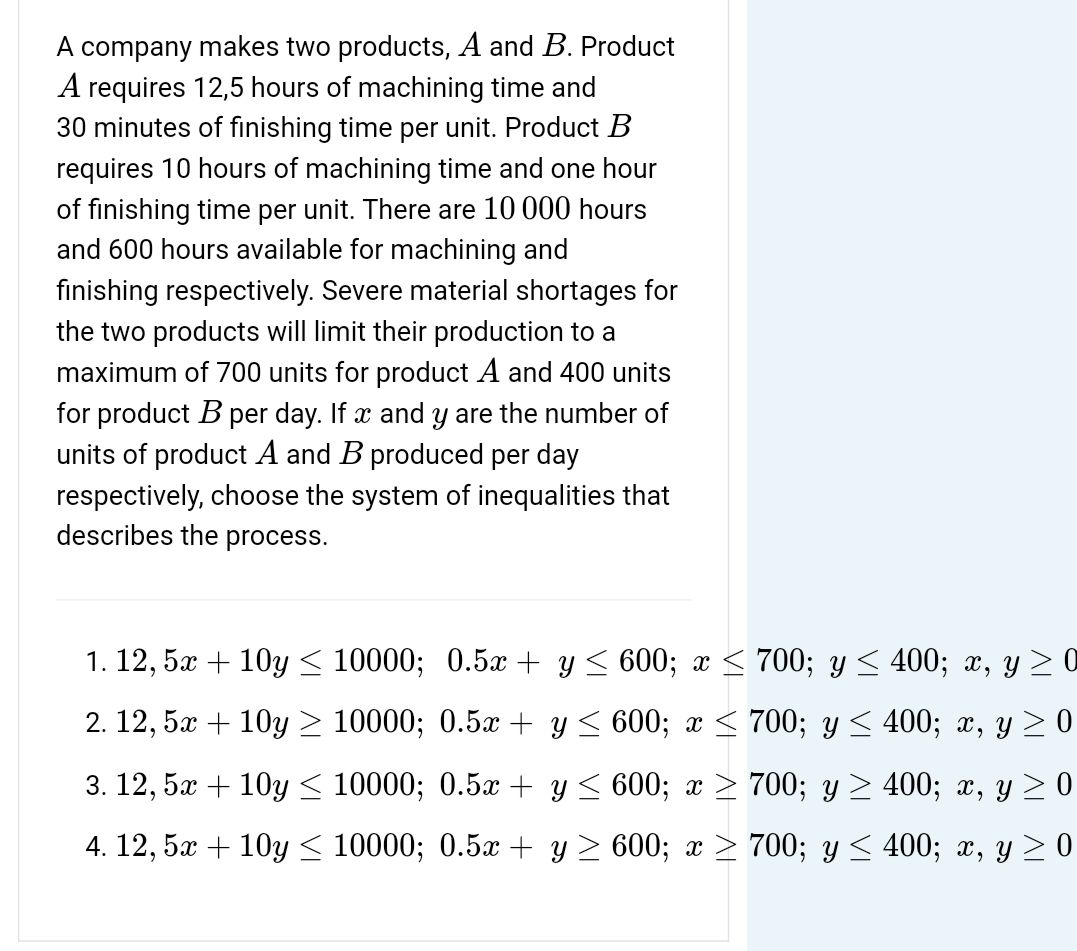### Still have math questions?

Algebra
QuestionA company makes two products, $$A$$ and $$B$$ . Product

$$A$$ requires $$12,5$$ hours of machining time and

$$30$$ minutes of finishing time per unit. Product $$B$$ requires $$10$$ hours of machining time and one hour of finishing time per unit. There are $$10000$$ hours and $$600$$ hours available for machining and finishing respectively. Severe material shortages for the two products will limit their production to a maximum of $$700$$ units for product $$A$$ and $$400$$ units for product $$B$$ per day. If $$x$$ and $$y$$ are the number of units of product $$A$$ and $$B$$ produced per day respectively, choose the system of inequalities that describes the process. 1. $$12,5 x + 10 y \leq 10000 ; 0.5 x + y \leq 600 ; x \leq 700 ; y \leq 400 ; x , y \geq 0$$

2. $$12,5 x + 10 y \geq 10000 ; 0.5 x + y \leq 600 ; x \leq 700 ; y \leq 400 ; x , y \geq 0$$

3. $$12,5 x + 10 y \leq 10000 ; 0.5 x + y \leq 600 ; x \geq 700 ; y \geq 400 ; x , y \geq 0$$

4. $$12,5 x + 10 y \leq 10000 ; 0.5 x + y \geq 600 ; x \geq 700 ; y \leq 400 ; x , y \geq 0$$